# Overview

The Random Forest Regression Forecast Processor can be used to calculate a forecast for a numeric column based on the random forest method.

A random forest is a meta estimator that fits a number of classifying decision trees on various sub-samples of the dataset and uses averaging to improve the predictive accuracy and control over-fitting.

`Note: Changed machine learning algorithm implementations in Spark 3 may slightly change results compared to Spark 2.`

# Input

The processor requires two input datasets. The first input port (the one on the left) corresponding to the training dataset (this data should be already labeled). The second input port (the one on the right) corresponding to the test dataset. Both datasets must contain a numerical variable column (The variable to predict).

# Output

The Random Forest Regression Forecast Processor returns three different output tables:

• Forecast Output: The original dataset with an additional column that contains the predicted values. The column name is specified in the third field in the processor's configuration.
• Mapping Output: Creates an RDD that contains all the categories for each feature that were mapped to fake values.
• Feature Importance Output: Returns the variable importance ranking for all independent variables within a two column table. It shows which of the independent variables were most important in predicting the dependent variable.

In addition, other outputs can be viewed within the "result" tab in the processor:

• RANDOM FOREST TREES: The different random trees used, along with each tree's weight.
• RANDOM FOREST INDEPENDENT IMPORTANCES: The bar chart for the different importance fractions assigned to each independent variable.
• RANDOM FOREST DEBUG: The generic JSON Object for the debug result.

# Example

In this example, the input dataset is the iris flower dataset. Each row of the dataset represents an iris flower, including its species and dimensions of its botanical parts, sepal and petal, in centimeters.

Here we're using the iris flower dataset in the other way around, which means the variety label here is one of the independent variable. The dependent variable to forecast is the petal_length.

## Workflow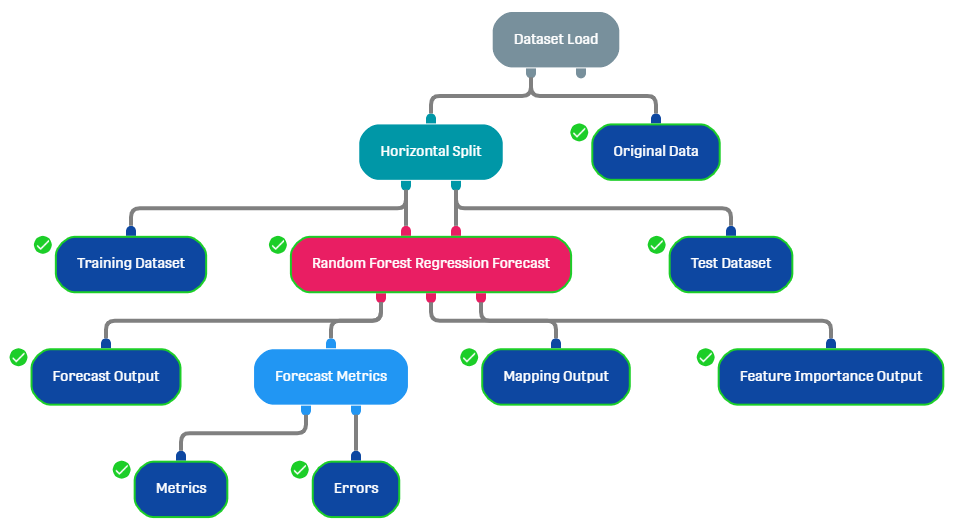The dataset is split into a training dataset (90%) and a test dataset (10%) using the Horizontal split processor. We also added a Forecast Metrics Processor to be able to evaluate the accuracy of our prediction.

## Result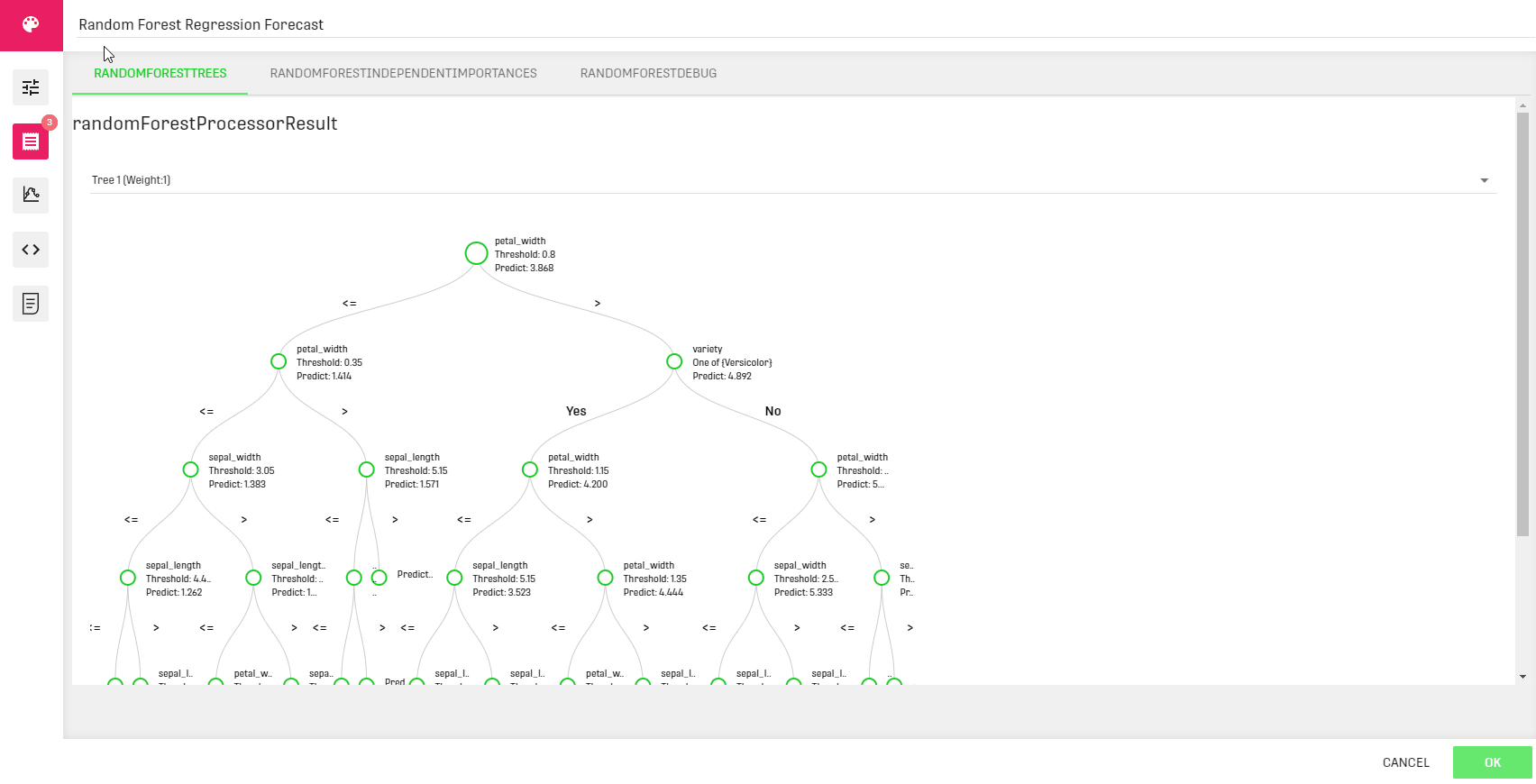Within the processor, the different decision trees used are displayed along with the corresponding weights.
A bar chart of the independent variables presenting the different importance fractions is also displayed in the second tab.

• Forecast Output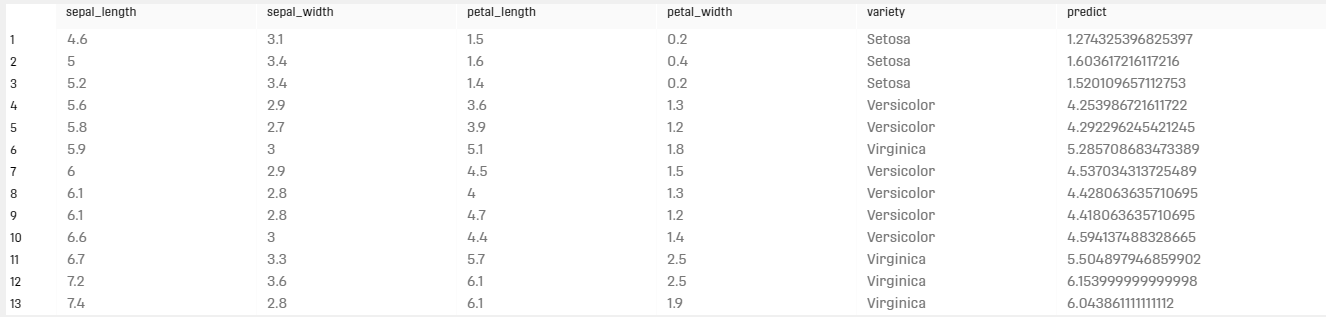• Feature Importance Output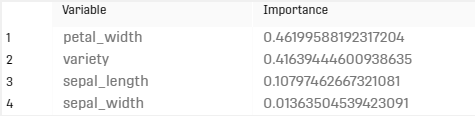• Forecast Metrics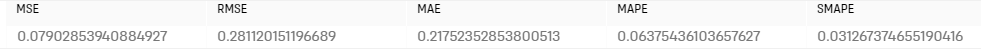# Related Articles

Random Forest Classification Forecast Processor